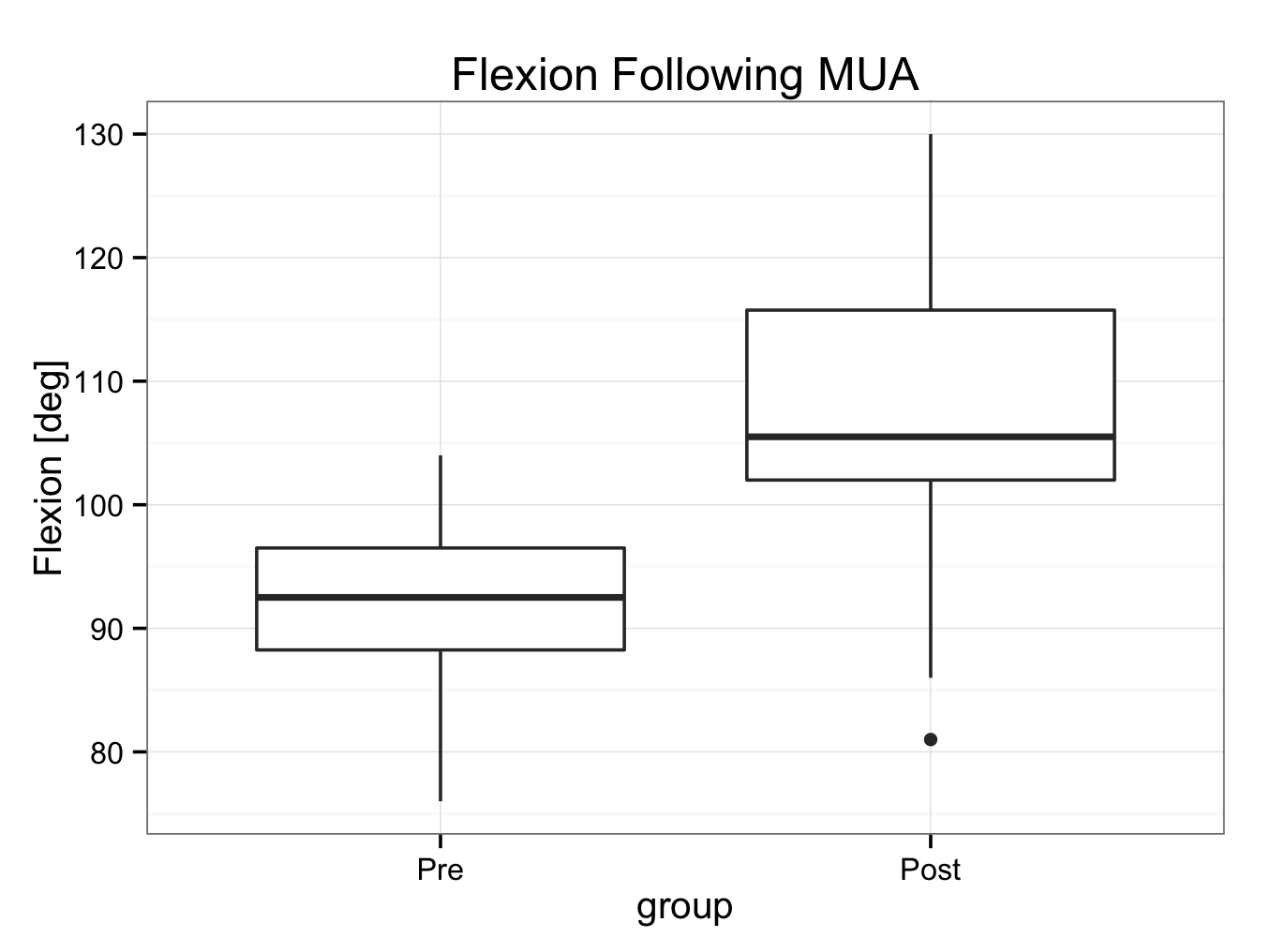# Box Plot

Box and whisker plots, or just box plots are used for the presentation of continuous data, that can be grouped in categories. The box represent the interquartile range and the horizontal line within it the median value. The whiskers represent the upper and lower adjacent values that lie within 1.5 times the interquartile range (although the definition may very between software programs). Any value outside the whiskers is marked separately as an ‘outlier’.

Download and open the plotbox.rda dataset for this example. The data set contains the maximum flexion in 20 patients before and after a manipulation under anaesthesia. The data can be shown by:

plotbox
Pre Post
1   94  115
2   95  103
3   89  113
4   89  122
5  101  103
6   76   93
7  102  118
8  104  102
9  103   81
10  93  102
11 101  124
12  92  130
13  77  103
14  95  125
15  89  108
16  95  106
17  92  105
18  84   89
19  83  113
20  86   86

To create a box and whisker plot of the pre-mua data:

pre<-ggplot()+geom_boxplot(aes(y=Pre,x=’PreMUA’),data=plotbox)
pre

Add a black and white theme, a title and axes labels:
pre<-pre+theme_bw()+ggtitle(‘Preoperative flexion’)+ylab(label=’max flexion [deg]’)+xlab(label=’Status’)
pre

If you are using ggplot < 0.9.2, the title can be set by:

pre<-pre+ opts(title=’Preoperative flexion’)
pre

To create a similar box and whisker plot of the post-mua flexion:

post<-ggplot()+geom_boxplot(aes(y=Post,x=’PostMUA’),data=plotbox)+theme_bw()+ggtitle(‘Postoperative flexion’)+ylab(label=’max flexion [deg]’)+xlab(label=’Status’)
post

If you are using ggplot < 0.9.2, the title can be set by:

post<-ggplot()+geom_boxplot(aes(y=Post,x=’PostMUA’),data=plotbox)+theme_bw()+opts(title=’Postoperative flexion’)+ylab(label=’max flexion [deg]’)+xlab(label=’Status’)
post

The preMUA plot looks symmetrical with the median in the middle of the box. However, the postMUA plot is clearly skewed as the median is located in the lower part of the box (right skewed, because the tail is to the ‘right’ or higher values). This is also reflected in the descriptive statistics:

descriptive.table(vars = d(Pre,Post),data= plotbox,
+ func.names =c(“Mean”,”Median”))
\$`strata: all cases `
Mean Median
Pre   92.00   92.5
Post 107.05  105.5

The mean and median are approximately the same in preMUA suggesting the data might be Normally distributed. However, postMUA this does not seem likely.

It would be nice to show both plots in one:

both<-ggplot()+geom_boxplot(aes(y=Pre,x=’Pre MUA’),data=plotbox)+geom_boxplot(aes(y=Post,x=’Post MUA’),data=plotbox)+theme_bw()+ggtitle(‘Flexion before and after MUA’)+ylab(label=’max flexion [deg]’)+xlab(label=’Status’)
both

If you are using ggplot < 0.9.2, the title can be set using:  opts(title=’Flexion before and after MUA’)

This will show the plot with the x-axis in alphabetical order. The easiest way to change this is by regrouping the data. Create a data-frame called ‘muapre’ for all preoperative data; the first collumn is called ‘flexion’ and the second ‘group’ (which are all “Pre”).  Similarly, a ‘muapost’ data-frame is created for all postoperative data with the same column names:
muapre<-data.frame(flexion=plotbox\$Pre,group=’Pre’)
muapre
flexion group
1       94   Pre
2       95   Pre
3       89   Pre
4       89   Pre
5      101   Pre
6       76   Pre
7      102   Pre
8      104   Pre
9      103   Pre
10      93   Pre
11     101   Pre
12      92   Pre
13      77   Pre
14      95   Pre
15      89   Pre
16      95   Pre
17      92   Pre
18      84   Pre
19      83   Pre
20      86   Pre

muapost<-data.frame(flexion=plotbox\$Post,group=’Post’)
muapost
flexion group
1      115  Post
2      103  Post
3      113  Post
4      122  Post
5      103  Post
6       93  Post
7      118  Post
8      102  Post
9       81  Post
10     102  Post
11     124  Post
12     130  Post
13     103  Post
14     125  Post
15     108  Post
16     106  Post
17     105  Post
18      89  Post
19     113  Post
20      86  Post

Now, bind the rows (rbind) together to create a new data-frame called mua:
mua<-rbind(muapre,muapost)
mua
flexion group
1       94   Pre
2       95   Pre
3       89   Pre
4       89   Pre
5      101   Pre
6       76   Pre
7      102   Pre
8      104   Pre
9      103   Pre
10      93   Pre
11     101   Pre
12      92   Pre
13      77   Pre
14      95   Pre
15      89   Pre
16      95   Pre
17      92   Pre
18      84   Pre
19      83   Pre
20      86   Pre
21     115  Post
22     103  Post
23     113  Post
24     122  Post
25     103  Post
26      93  Post
27     118  Post
28     102  Post
29      81  Post
30     102  Post
31     124  Post
32     130  Post
33     103  Post
34     125  Post
35     108  Post
36     106  Post
37     105  Post
38      89  Post
39     113  Post
40      86  Post

Now the ‘mua’ data-frame can be used to create a grouped box and whisker plot:

muaplot<-ggplot() + stat_boxplot(aes(y = flexion,x = group),data=mua) +theme_bw() + ggtitle(label = ‘Flexion Following MUA’) + ylab(label = ‘Flexion [deg]’)
muaplot

If you are using ggplot < 0.9.2, the title can be set using: opts(title=’Flexion Following MUA’)